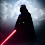## Friday, July 1, 2011

### Equity Valuation Based on GDP Growth

An update (with a small addition) of a post from May 2010

Step 1) Take the S&P 500 Index (lots of data here) and divide that level by the current level of nominal GDP (you can find that here).

Below is a chart of just that going back to 1951 and the corresponding 60 year average.Step 2)

Fair Value Methodology: Take that 60 year average (8.25%) to normalize the first year of your 'Fair Value S&P 500' "FV" Index by taking nominal GDP at the starting date (in this case June 1951 = \$336.6 billion) and multiplying by the percent (x 8.25% = 27.77). In this case 27.77 = the FV Index level (or the starting value normalized).

Matched Starting Value Methodology: Simply start the index at the value of the S&P 500 as of June 1951 = 21.55.

Step 3) Using that 27.77 (or calculated value using a different time frame) as the FV Index starting value or 21.55 as the matched starting value, at each interval increase the index by the change in nominal GDP (note... in the chart below, I estimated the Q2 GDP at 2.0% annualized). Why nominal GDP? Go here.

The below chart shows these calculated indices vs the actual S&P 500 index.Step 4)
Calculate the percent the S&P 500 Index is over or under valued relative to each calculated index.Relationship (returns are annualized)....Note 1: under these methodologies, the S&P 500 is currently at or above fair value, still implying a decent ten year forward change in the S&P 500 plus dividends minus inflation.
Note 2: a change in the starting value of the FV Index would simply shift the x-axis to the right or left (i.e. it would not change the relationship between the two)

Source: Irrational Exuberance

1.Wow! That was tons of work, thanks Jake and have a great 4th of July weekend.

2.you as well!

enjoy the fishing season

3.1. Fascinating stuff - S&P/GDP seems like a nice complement to Tobin's Q and S&P/NIPA profits

2. Is is possible to attach a label with the current value for each of the methodologies in charts 2 and 3? I can guess, but my vision ain't what it used to be....

Many thanks for sharing!